Question

# The wavefunction for a traveling wave is given by y(x,t)= 4.5 sin (1.7x -8.2t+0.35). what is...

The wavefunction for a traveling wave is given by y(x,t)= 4.5 sin (1.7x -8.2t+0.35). what is the period of the wave?

a/ 1.73

b/ 8.20

c/ 0.77

d/ 0.55

e/ 1.54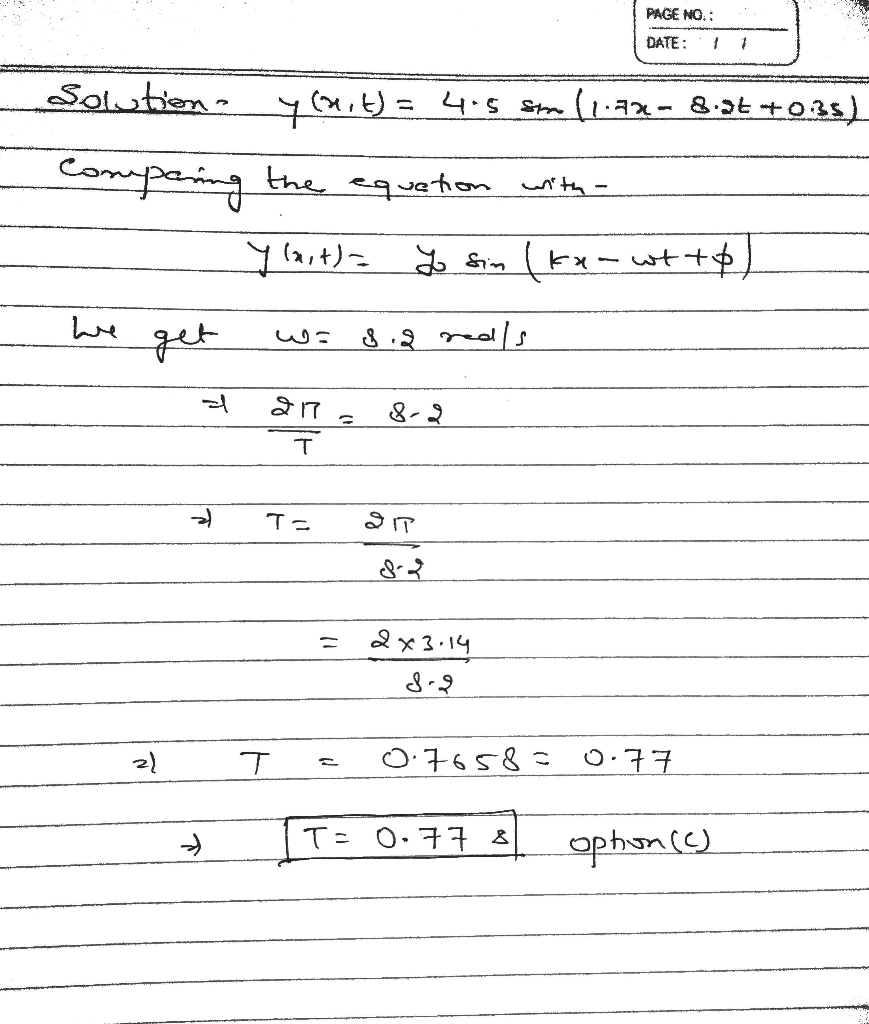If you have any doubt please write in the comment box or else Please like thank you

#### Earn Coins

Coins can be redeemed for fabulous gifts.

Similar Homework Help Questions
• ### A traveling wave is described by the equation: TT y(x, t) = (0.35 cm) sin (...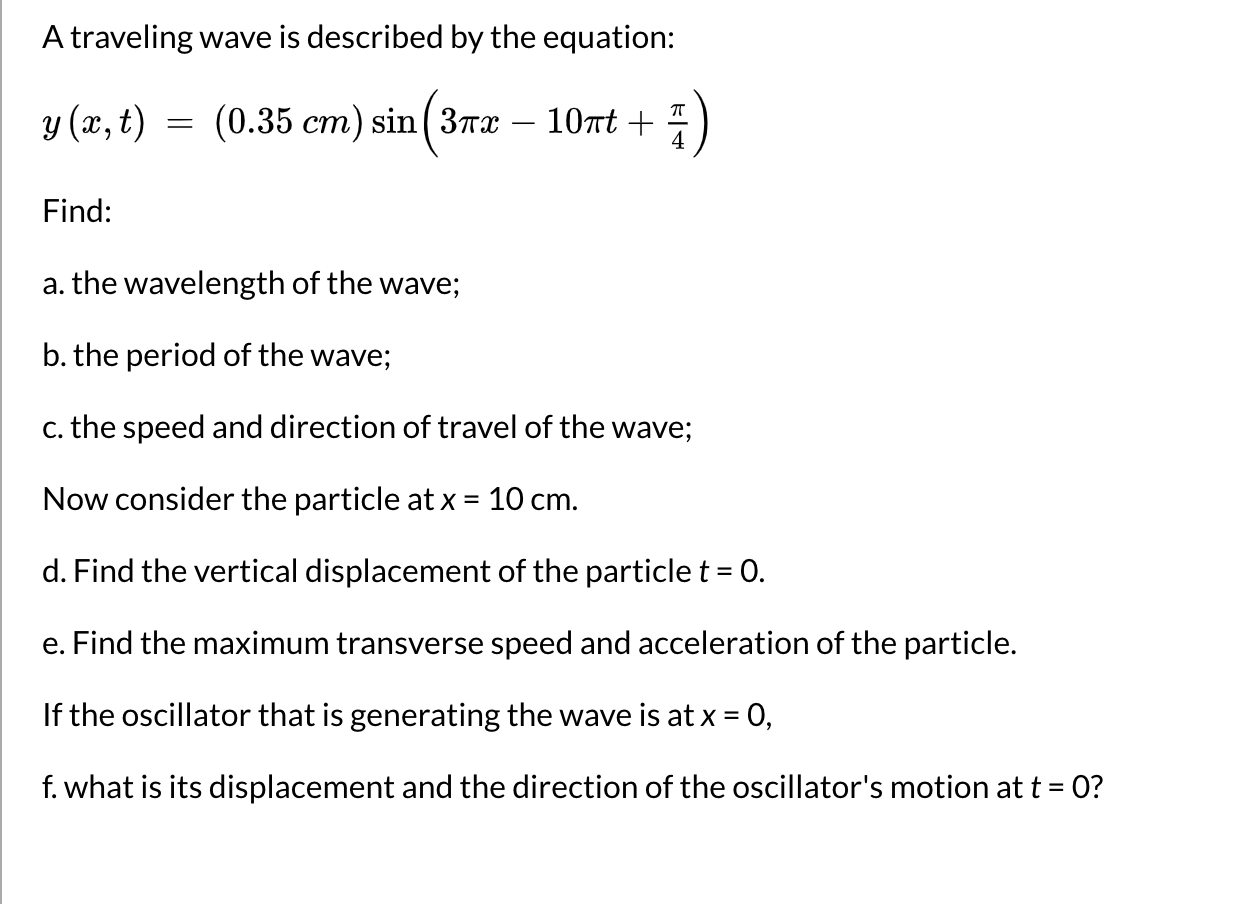A traveling wave is described by the equation: TT y(x, t) = (0.35 cm) sin ( 3173 107t + 4 Find: a. the wavelength of the wave; b. the period of the wave; c. the speed and direction of travel of the wave; Now consider the particle at x = 10 cm. d. Find the vertical displacement of the particle t = 0. e. Find the maximum transverse speed and acceleration of the particle. If the oscillator that is generating...

• ### The transverse diaplacement for a wave on a string traveling in the +x direction is y(x,t)...

The transverse diaplacement for a wave on a string traveling in the +x direction is y(x,t) = (0.0090) sin (66.8m^-1)x-(310s^-1)t) what is it’s a) velocity b)wavelength c)frequency d)period e)amplitude (in units)

• ### What are the parts of this traveling wave? y(x,t) = (9.00 m) sin( (67 m-')x +...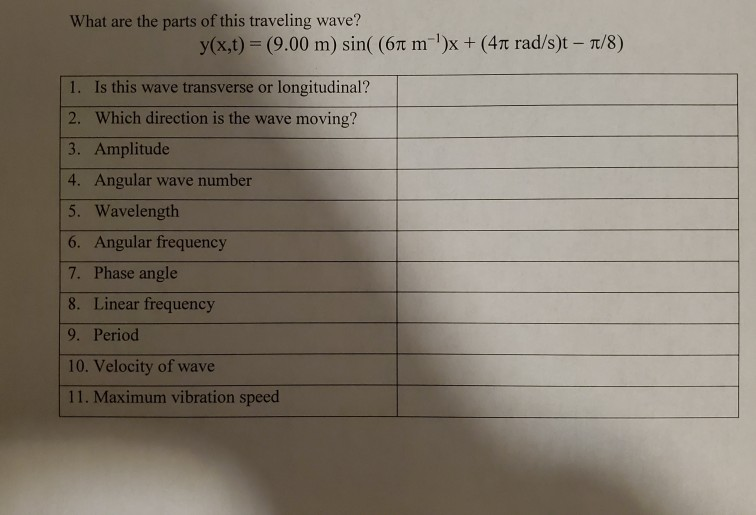What are the parts of this traveling wave? y(x,t) = (9.00 m) sin( (67 m-')x + (41 rad/s)t – 7/8) 1. Is this wave transverse or longitudinal? 2. Which direction is the wave moving? 3. Amplitude 4. Angular wave number 5. Wavelength 6. Angular frequency 7. Phase angle 8. Linear frequency 9. Period 10. Velocity of wave 11. Maximum vibration speed

• ### The wave function for a traveling wave on a taut string is (in SI units) y(x,t)...

The wave function for a traveling wave on a taut string is (in SI units) y(x,t) = 0.360 sin 14πt − 2πx + π 4 (a) What are the speed and direction of travel of the wave? speed m/s direction (b) What is the vertical position of an element of the string at t = 0, x = 0.200 m? m (c) What is the wavelength of the wave? m (d) What is the frequency of the wave? Hz (e)...

• ### If y(x, t) = (5.4 mm)sin(kx + (575 rad/s)t + ϕ) describes a wave traveling along...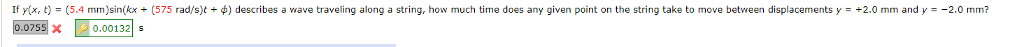If y(x, t) = (5.4 mm)sin(kx + (575 rad/s)t + ϕ) describes a wave traveling along a string, how much time does any given point on the string take to move between displacements y = +2.0 mm and y = −2.0 mm? If y(x, t) = (5.4 mm)sin(kx + (575 rad/s)t + φ) describes a wave traveling along a string, how much time does any given point on the string take to move between displacements y = +2.0 mm and...

• ### The equation of a transverse wave traveling along a string is y = (0.11 m)sin[(0.78 rad/m)x...

The equation of a transverse wave traveling along a string is y = (0.11 m)sin[(0.78 rad/m)x - (14 rad/s)t] (a) What is the displacement y at x = 2.6 m, t = 0.27 s? A second wave is to be added to the first wave to produce standing waves on the string. If the wave equation for the second wave is of the form y(x,t) = ymsin(kx + ωt), what are (b) ym, (c) k, and (d) ω (e) the...

• ### The equation of a transverse wave traveling along a string is y = 0.419 sin(0.265x -...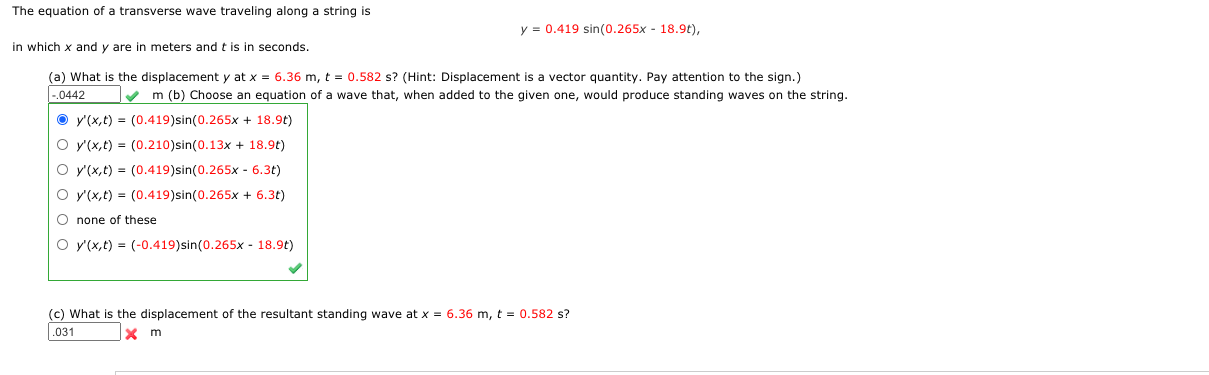The equation of a transverse wave traveling along a string is y = 0.419 sin(0.265x - 18.90), in which x and y are in meters and t is in seconds. (a) What is the displacement y at x = 6.36 m, t = 0.582 s? (Hint: Displacement is a vector quantity. Pay attention to the sign.) -.0442 m(b) Choose an equation of a wave that, when added to the given one, would produce standing waves on the string. O V'(x,t)...

• ### 13. I The displacement of a wave traveling in the positive x-direction is D(x, t) (3.5 cm) sin(2....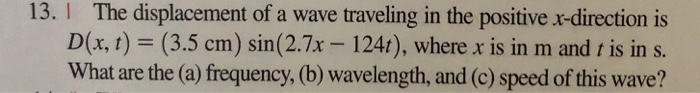13. I The displacement of a wave traveling in the positive x-direction is D(x, t) (3.5 cm) sin(2.7x 1241), where x is in m and t is in s. What are the (a) frequency, (b) wavelength, and (c) speed of this wave? 13. I The displacement of a wave traveling in the positive x-direction is D(x, t) (3.5 cm) sin(2.7x 1241), where x is in m and t is in s. What are the (a) frequency, (b) wavelength, and (c)...

• ### The equation of a transverse wave traveling along a string is y (0.24 m)sin[(0.87 rad/m)x (14...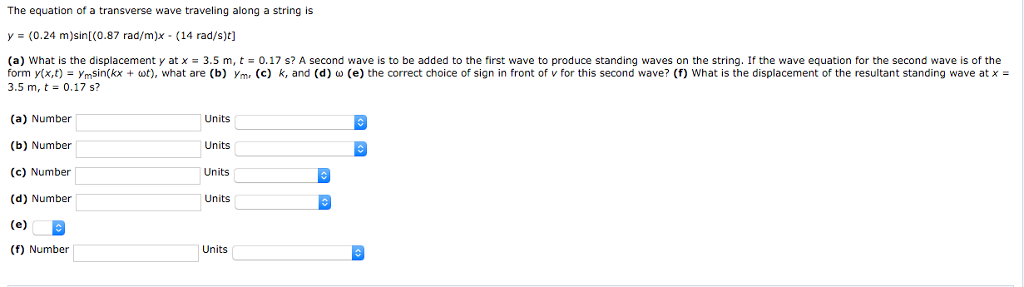The equation of a transverse wave traveling along a string is y (0.24 m)sin[(0.87 rad/m)x (14 rad/s)t] (a) what is the displacement y at x ? 3.5 m, t 0.17 s? A second wave is to be added to the first wave to produce standing waves on the string. If the wave equation for the second wave is of the form y(x,t)-ymsin(kx + at), what are (b) ym, (c) k, and (d) ? (e) the correct choice of sign in...

• ### 4) The given wave is traveling in a rope to the right. t = 1 second...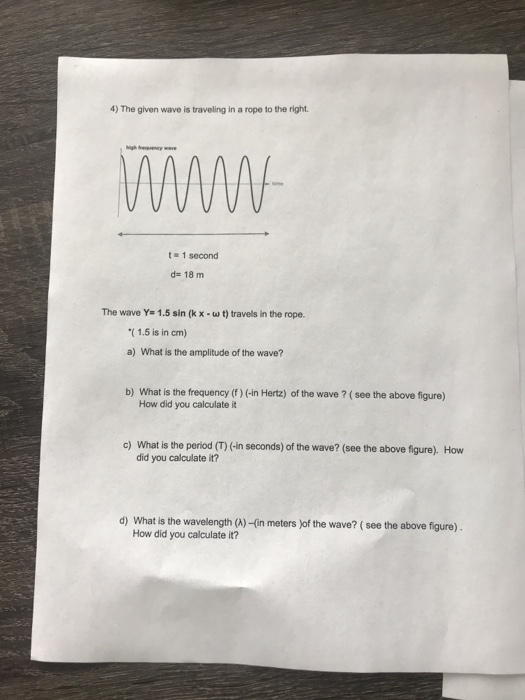4) The given wave is traveling in a rope to the right. t = 1 second de 18 m The wave Y= 1.5 sin(kx-wt) travels in the rope. *(1.5 is in cm) a) What is the amplitude of the wave? b) What is the frequency (f) (-in Hertz) of the wave ? ( see the above figure) How did you calculate it c) What is the period (T) (-in seconds) of the wave? (see the above figure). How did you...